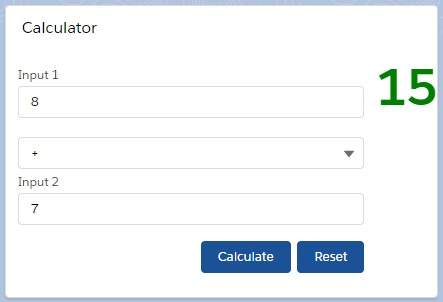# Import/Export JavaScript file in LWC

In ES6 JS architecture, we can export the variables or functions in JS file, so other module can use.

Here, we will create simple calculator using import/export functionality in Lightning Web Component framework. Let’s see.

Step 1: First, we are going to create a LWC named “calculator” where we will define only JS file that is “calculator.js”.

calculator.js

```const calculator = (first_param, second_param, mode) => {
if (mode === "add" || mode === "+" || mode === "Add" || mode === "ADD") {
return +first_param + +second_param;
}

if (
mode === "subtraction" ||
mode === "-" ||
mode === "Subtraction" ||
mode === "SUBTRACTION"
) {
return first_param - second_param;
}

if (
mode === "divide" ||
mode === "/" ||
mode === "Divide" ||
mode === "DIVIDE"
) {
return first_param / second_param;
}

if (
mode === "multiplication" ||
mode === "*" ||
mode === "Multi" ||
) {
return first_param * second_param;
}
return 0;
};

export { calculator };

```

Here we have defined a function named “calculator” in which there are three parameters such as first_param, second_param and mode which denotes the operation type of calculation.

At last, we have explicitly exported the function as calculator so other LWC can import it.

Step 2: Next, we will create a LWC named “calculation” which will display the result.

So, we need to update the calculation.js-meta.xml to set the visibility in Lightning App Builder.

calculation.js-meta.xml

```<?xml version="1.0" encoding="UTF-8"?>
<apiVersion>45.0</apiVersion>
<isExposed>true</isExposed>
<targets>
<target>lightning__AppPage</target>
<target>lightning__RecordPage</target>
<target>lightning__HomePage</target>
</targets>
</LightningComponentBundle>
```

Then, we will write calculation.html file.

calculation.html

```<template>
<lightning-card title="Calculator">

<div class="slds-float_right">
<label class="resClass">{result}</label>
</div>

<lightning-layout>
<lightning-input type="number" label="Input 1 " value={input1} onchange={changeInput1}></lightning-input>
<lightning-combobox label="" value={mode} options={modeOptions} onchange={changeMode}></lightning-combobox>
<lightning-input type="number" label="Input 2 " value={input2} onchange={changeInput2}></lightning-input>

<div class="slds-float_right slds-m-top_medium">
<lightning-button variant="brand" label="Calculate" onclick={doCalculation}></lightning-button>
&nbsp;&nbsp;
<lightning-button variant="brand" label="Reset" onclick={doReset}></lightning-button>
</div>

</lightning-layout-item>
</lightning-layout>
</lightning-card>
</template>
```

Then, we need to update calculation.js file.

calculation.js

```import { LightningElement, track } from 'lwc';
import { calculator } from 'c/calculator';

export default class Calculation extends LightningElement {

@track result = "";
@track input1;
@track input2;
@track mode;

modeOptions = [
{ label: "+", value: "+" },
{ label: "-", value: "-" },
{ label: "*", value: "*" },
{ label: "/", value: "/" }
];

changeInput1(event) {
this.input1 = event.target.value;
}

changeInput2(event) {
this.input2 = event.target.value;
}

changeMode(event) {
this.mode = event.target.value;
}

doCalculation() {
/*eslint-disable-next-line*/
console.log("input1--->" +this.input1 + "input2---->" +this.input2 +"mode--->" +this.mode);
this.result = calculator(this.input1, this.input2, this.mode);
}

doReset(){
this.input1 = null;
this.input2 = null;
this.mode = null;
}
}
```

Here, you can see we have imported the function calculator from “calculator.js” file. In doCalculation() function, we have invoked calculator function from calculator.js using three attributes.

At last, we have defined calculator.css file to beautify the output.

calculator.css

```.resClass {
color: green;
font-weight: bold;
font-size: 50px;
}
```

ResultSo, here we have given the first param as 8, second param as 7, mode of operation as “+” and we will get the result as summation of two integers as 15 in Lightning Web Component through calculator.js file.

42,819 total views, 24 views today